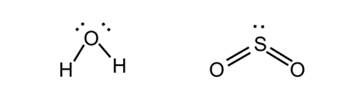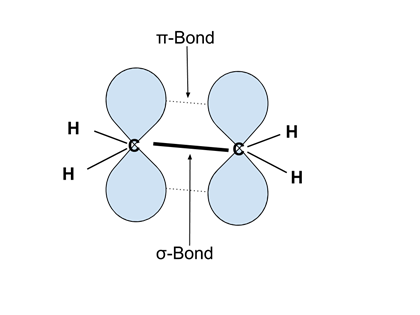# Enduring Understanding 2.C.4: Lewis Structures and VSEPR

• The electronic structure of molecules can be illustrated by Lewis structures, which can be used to and properties such as geometry, bond orders, bond lengths, relative bond energies, and dipoles.

• Examples: Lewis structures of H2O and SO2:
• <• Valence Shell Electron Pair Repulsion (VSEPR) theory, along with Lewis structures can be used to predict molecular geometry. It assumes that bonds and lone pairs repel each other, and will arrange themselves to be as far from each other as possible. The following are the geometries that will be adopted by atoms with n atoms/lone pairs around them:

• 2: Linear (e.g. HCN), bond angle 180°
• 3: Trigonal Planar (BF3), bond angle 120°
• 4: Tetrahedral (CH4), bond angle 109.5°
• 5: Trigonal Bipyramidal (PCl5), bond angles 90°, 120°
• 6: Octagonal (SF6), bond angle 90°
• In the example Lewis structures drawn above, H2O has four bonds/lone pairs around it and therefore adopts a tetrahedral geometry. SO2 has three, and therefore is trigonal planar. Considering the atoms (and not the lone pairs), they are therefore both 'bent', with a bond angle around 109.5° (H2O) and 120° (SO2).

• Atoms in molecules (especially carbon) are often described as being hybridized - referring to the atomic orbitals that are involved in forming the bonding orbitals. Three examples:
• sp hybridized: molecule is linear, bond angle 180°
• sp2 hybridized: molecule is trigonal planar, bond angle 120°
• sp3 hybridized: molecule is tetrahedral, bond angle 109.5°

• Bonds can be referred to as sigma (σ) or pi (π). σ-bonds have maximum electron density in the plane of the two bonded atoms. π bonds have a node (no electron density) in the plane of the bonded atoms.
• σ-bonds have better overlap and are stronger than π bonds.
• Rotation around σ-bonds is possible, but not around π bonds. This leads to structural isomers, for example in disubstituted alkenes like cis- and trans-2-butene.

• Example: The image below illustrates ethylene (C2H4), with the σ-bond between the two carbons as a solid dark line, and the π bond above and below the HC-CH plane illustrated by the overlap of the two p orbitals in blue. Note that rotation around the C-C bond is not possible, as this would destroy the overlap of the two p orbitals and thereby break the bond.
• <Related Links: Chemistry Chemistry Quizzes AP Chemistry Notes Ionic and Metallic Bonding

To link to this Lewis Structures and VSEPR page, copy the following code to your site: